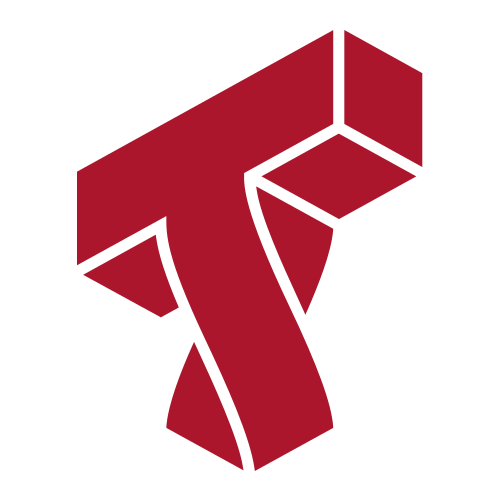# Lecture 18: Clustering¶

### Applied Machine Learning¶

Volodymyr Kuleshov
Cornell Tech

# Part 1: Gaussian Mixture Models¶

Clustering is a common unsupervised learning problem with numerous applications.

We will start by defining the problem and outlining some models for this problem.

# Review: Unsupervised Learning¶

We have a dataset without labels. Our goal is to learn something interesting about the structure of the data:

• Clusters hidden in the dataset.
• Outliers: particularly unusual and/or interesting datapoints.
• Useful signal hidden in noise, e.g. human speech over a noisy phone.

# Review: Unsupervised Learning¶

We will assume that the dataset is sampled from a probability distribution $P_\text{data}$, which we will call the data distribution. We will denote this as $$x \sim P_\text{data}.$$

The dataset $\mathcal{D} = \{x^{(i)} \mid i = 1,2,...,n\}$ consists of independent and identicaly distributed (IID) samples from $P_\text{data}$.

# Clustering¶

Clustering is the problem of identifying distinct components in the data distribution.

• A cluster $C_k \subseteq \mathcal{X}$ is associated with a subset of the $x$ coming from $P_\text{data}$.
• Datapoints in a cluster are more similar to each other than to other clusters
• Clusters are usually defined by their centers, and potentially by other shape parameters.

# Review: $K$-Means¶

$K$-Means is the simplest example of a clustering algorithm.

• The algorithm seeks to find $K$ hidden clusters in the data.
• Each cluster is characterized by its centroid (its mean).
• The clusters reveal interesting structure in the data.

We seek centroids $c_k$ such that the distance between the points and their closest centroid is minimized: $$J(\theta) = \sum_{i=1}^n || x^{(i)} - \text{centroid}(f_\theta(x^{(i)})) ||,$$ where $\text{centroid}(k) = c_k$ denotes the centroid for cluster $k$.

This is best illustrated visually (from Wikipedia):